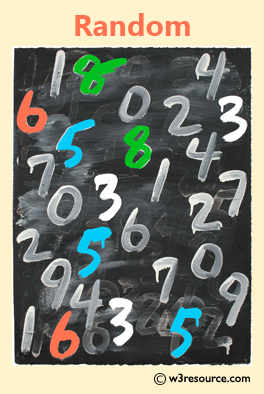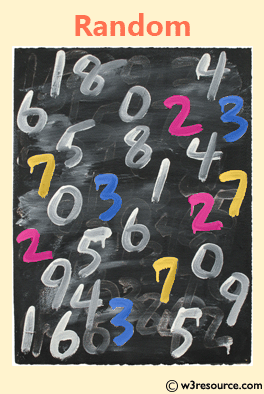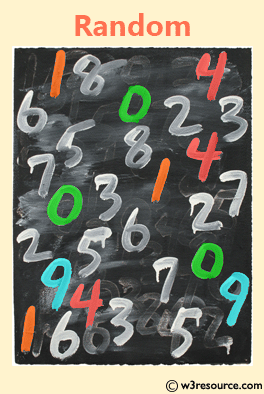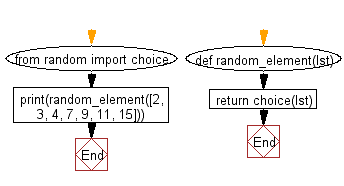﻿ Python: Select an item randomly from a list - w3resource# Python: Select an item randomly from a list

## Python List: Exercise - 25 with Solution

Write a Python program to select an item randomly from a list.

Use random.choice() to get a random element from a given list.

Example - 1 :Example - 2 :Example - 3 :Sample Solution-1:

Python Code:

``````import random
color_list = ['Red', 'Blue', 'Green', 'White', 'Black']
print(random.choice(color_list))
```
```

Sample Output:

```Black
```

## Visualize Python code execution:

The following tool visualize what the computer is doing step-by-step as it executes the said program:

Sample Solution-2:

Python Code:

``````from random import choice
def random_element(lst):
return choice(lst)
print(random_element([2, 3, 4, 7, 9, 11, 15]))
```
```

Sample Output:

```4
```

Flowchart:## Visualize Python code execution:

The following tool visualize what the computer is doing step-by-step as it executes the said program:

Python Code Editor:

Have another way to solve this solution? Contribute your code (and comments) through Disqus.

What is the difficulty level of this exercise?

Test your Python skills with w3resource's quiz

﻿

## Python: Tips of the Day

Floor Division:

When we speak of division we normally mean (/) float division operator, this will give a precise result in float format with decimals.

For a rounded integer result there is (//) floor division operator in Python. Floor division will only give integer results that are round numbers.

```print(1000 // 300)
print(1000 / 300)```

Output:

```3
3.3333333333333335```